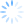Using our Microsoft Excel add-in, CDXZipStream, it’s easy to find the zip codes within a radius distance of a target zip.  Just right-click on a worksheet cell, and using the function CDXRadius, input the target zip code and specified radius distance;  an array or text string listing the zip codes within the radius will be returned to the worksheet.  However, this only applies to a straight-line distance around the target.  What if you need zip codes within a specified driving distance or time?

CDXZipStream does not have a special function for this type of calculation, but you can use a combination of two functions, CDXRadius and CDXRouteMP, to easily get the desired result.  For example, let’s say we’re looking for the zip codes within a 20-mile driving distance of zip code 07869.  (We can also do this for driving time as well – we’ll talk about that case later in this article.)  The first step is to find the zip codes within a somewhat larger straight-line radius, using CDXRadius  For this example we’ll use 25 miles.  This will capture all the zip codes within the 20-mile driving radius, and then some.  So the input for CDXRadius is:Once we have the returned list of zip codes from CDXRadius, we’ll use CDXRouteMP to calculate the driving distance between each of these zips and our target zip.  Again, we right-click on the worksheet, select CDXRouteMP, and use this following input to calculate the driving distance to 07869:Note that the first returned zip code from CDXRadius (other than the target zip in the first row) is in worksheet cell A2.  We use this as the second address in the calculation, so when we copy the resulting formula it will apply to all zip codes in each row.  The formula we obtain from this is:

=CDXRouteMP(1,0,"07869",A2)

We can copy this formula all along the list in Column C to get all the driving distances for all zip codes.  Columns A and B show the zip codes and their straight-line distances from the target, as calculated by CDXRadius, and colums C is the calculated driving distance from CDXRouteMP.   The first few rows are shown here.Now just use Excel’s sort or auto-filter to find zip codes within a 20-mile driving distance.  When using auto-filter, select a “Number Filter” of “Less than” 20 for column C, and only those rows with distances of 20 miles or less will be visible in the worksheet.

What if you want to find a radius based on driving time?  The process is very similar, but when using the CDXRadius function assume that the vehicle is travelling 90 miles an hour (or 1.5 miles per minute) to obtain the initial list of zip codes.  For a 30-minute driving time, this means the specified (straight-line) radius distance would be 1.5 x 30 or 45 miles.  You can then narrow down the list obtained from CDXRadius by using the CDXRouteMP function to calculate driving time:As we did before, sort or auto-filter to obtain only those zip codes within a 30-minute driving time.

If you would like a map of the result, use CDXRadius to output a drive time map to the worksheet:To see short tutorials about the functions CDXRouteMP and CDXDistance, please refer to the following:

Find Zip Codes in a Radius Using Excel

Driving Distance Calculator in Excel